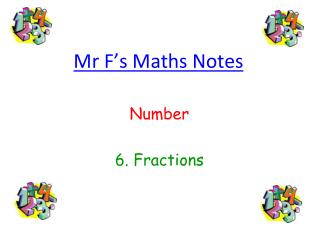# Mr F’s Maths Notes - PowerPoint PPT PresentationDownload PresentationMr F’s Maths Notes

Mr F’s Maths Notes
Download Presentation## Mr F’s Maths Notes

- - - - - - - - - - - - - - - - - - - - - - - - - - - E N D - - - - - - - - - - - - - - - - - - - - - - - - - - -
##### Presentation Transcript

1. Mr F’s Maths Notes Number 6. Fractions

2. Things you might need to be able to do with fractions… This will vary with your ageand whatmaths setyou are in, but here is a list of some of the things you might need to be able to do with fractions: 1. Know the all important fraction’s lingo 2. Know how to find the fraction of a quantity 3. Know all about equivalent fractions so you can simplify 4.Understand proper and improper fractions 5. Know how to add, subtract, multiply and divide using decimals 6. Understand the relationship between fractions, decimals and percentages And so, without further ado, let’s get on with it… 6. Fractions 1. Fraction’s Lingo Right, let’s try and stop talking about the top and the bottom of a fraction, and instead go for something a bit fancier, like this: numerator Warning: every now and again, Mr F forgets to call them this, so watch out for “top” and “bottom” creeping into these notes denominator

3. 2. Fraction of a Quantity There is a simple method which always works with these types of questions: 1.Divide by the bottom (finds you the value of one fraction) 2.Multiply by the top (gives you the value of the number of fractions you need) Example 1 Example 2 What is of 24? Find of 2.436 Kg (give your answer in grams) 1. If you divide the quantity (24) by the denominator of the fraction (4), it tells you the value of Now, if before we start, let’s change 2.436 Kg into grams so we get nicer numbers and so we are ready for our answer: so, 2.436kg = 2436g so, Now we just do the same as before: 1.Divide by the bottom (2436 ÷ 7) 2.Multiply our answer by the top ( x 5 ) 2. But we don’t want , we want so, we must multiply our answer (6) by the numerator (3) so, so, Remember: give units in your answer: 1740g

4. 3. Equivalent Fractions Equivalent fractions are just fractions which have exactly the same value. You need good knowledge of equivalent fractions when simplifying your answers, and also when adding and subtracting fractions. Here is the rule: Whatever you multiply or divide the top by, do the exact same to the bottom! Example 1 Example 2 Example 3 Simplify: We are looking to make the fraction as simple as possible (i.e. contain the smallest possible whole numbers) We need a number to divide both the top and the bottom by (a factor of both) We stop dividing when the top and the bottom do not share any more factors It doesn’t matter how long it takes! Ask yourself: “what has been done to the 7 to make it 21?” And then do the same to the top! Ask yourself: “what has been done to the 49 to make it 7?” And then do the same to the bottom! x 3 ÷ 7 ÷ 2 ÷ 3 x 3 ÷ 7 ÷ 2 ÷ 3

5. 4. Proper and Improper Fractions In a proper fraction, the bottom is bigger than the top, like: In an improper fraction (top heavy), the top is bigger than the bottom, like: Sometimes, improper fractions are written as mixed number fractions, like: You need to be able to switch between improper and mixed number fractions! Example 1 Example 2 Write: as a mixed number fraction Write: as an improper fraction Okay, so here we have 22 lots of Right, now we have 3 whole ones, and 5 lots of How many do we need to make one whole? How many lots of are then in each whole?... Well, if you think about a cake sliced into fifths, then we would need 5 slices to make a whole Well, one whole is , so there must be 8! So, how many wholes can we make out of our 22? So, how many lots of in our 3 wholes? Well, 5 goes into 22… erm… 4 times, with a remainder of… erm… erm… 2! 3 x 8 = 24! But remember, we also have our 5 lots of So, our 22 makes 5 wholes with 2 parts left over So, altogether we have (24 + 5) lots of So… So…